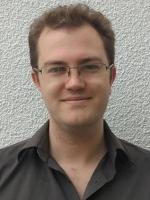# Department of Pure Mathematics and Mathematical StatisticsProfessor of Mathematics

Research Interests: Algebraic and Geometric Topology, in particular: mapping class groups, moduli spaces, cobordism categories, spaces of manifolds, surgery, configuration spaces, characteristic classes, K-theory and applications of homotopy theory to geometry.

## Publications

Tautological rings and stabilisation
O Randal-Williams
– Glasgow Mathematical Journal
(2021)
1
An ${\bf H}P^2$-bundle over $S^4$ with nontrivial $\hat{A}$-genus
M Krannich, A Kupers, O Randal-Williams
– Comptes Rendus. Mathématique
(2021)
359,
149
A vanishing theorem for tautological classes of aspherical manifolds
F Hebestreit, M Land, W Lück, O Randal-Williams
– Geometry and Topology
(2021)
25,
47
Mapping class groups of simply connected high-dimensional manifolds need not be arithmetic
M Krannich, O Randal-Williams
– Comptes Rendus Mathematique
(2020)
358,
469
ON the COHOMOLOGY of TORELLI GROUPS
A Kupers, O Randal-Williams
– Forum of Mathematics, Pi
(2020)
8,
e7
Exposé Bourbaki 1164 : Homology of Hurwitz spaces and the Cohen-Lenstra heuristic for function fields (after Ellenberg, Venkatesh, and Westerland)
O Randal-Williams
– Astérisque
(2020)
422,
469
The cohomology of Torelli groups is algebraic
A Kupers, O Randal-Williams
– Forum of Mathematics, Sigma
(2020)
8,
e64
Semi-simplicial spaces
J Ebert, O Randal-Williams
– Algebraic and Geometric Topology
(2019)
19,
2099
$E_2$-cells and mapping class groups
S Galatius, A Kupers, O Randal-Williams
– Publications Mathematiques de l'Institut des Hautes Etudes Scientifiques
(2019)
130,
1
Moduli spaces of manifolds: a user's guide
S Galatius, O Randal-Williams
(2019)
• 1 of 5
• >

E2.06

01223 764291